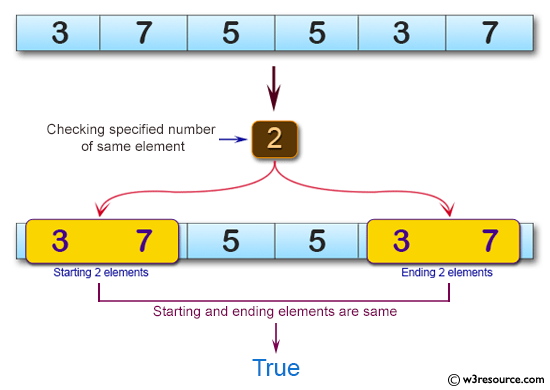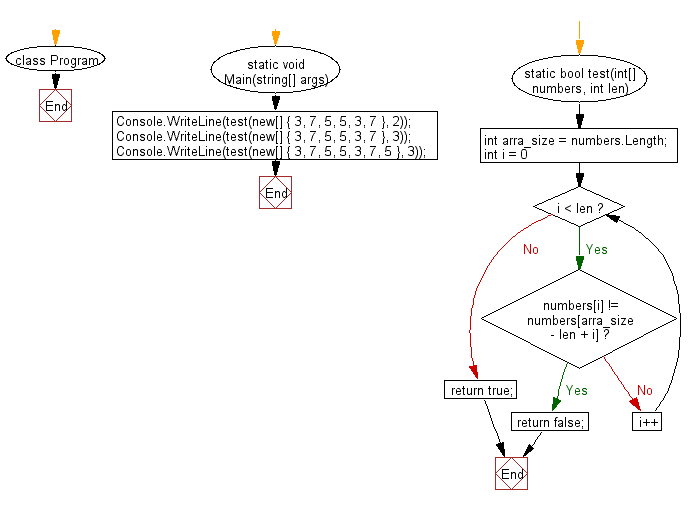﻿ C# - Check if a value appears at the start, end of an array# C# Sharp Basic Algorithm Exercises: Check a given array of integers and return true if the specified number of same elements appears at the start and end of the given array

## C# Sharp Basic Algorithm: Exercise-125 with Solution

Write a C# Sharp program to check a given array of integers and return true if the specified number of same elements appears at the start and end of the given array.

Pictorial Presentation:Sample Solution:

C# Sharp Code:

``````using System;
namespace exercises
{
class Program
{
static void Main(string[] args)
{
Console.WriteLine(test(new[] { 3, 7, 5, 5, 3, 7 }, 2));
Console.WriteLine(test(new[] { 3, 7, 5, 5, 3, 7 }, 3));
Console.WriteLine(test(new[] { 3, 7, 5, 5, 3, 7, 5 }, 3));

}

static bool test(int[] numbers, int len)
{
int arra_size = numbers.Length;

for (int i = 0; i < len; i++)
{
if (numbers[i] != numbers[arra_size - len + i])
{
return false;
}
}

return true;
}
}
}
```
```

Sample Output:

```True
False
True
```

Flowchart:C# Sharp Code Editor:

Improve this sample solution and post your code through Disqus

What is the difficulty level of this exercise?

Test your Programming skills with w3resource's quiz.

﻿